RANDOM Statement

RANDOM random-effect ~distribution SUBJECT=variable <options> ;

The RANDOM statement defines a single random effect and its prior distribution or an array of random effects and their prior distribution. The random-effect must be represented by either a symbol or an array. The RANDOM statement must consist of the random-effect, , a tilde (~), the distribution for the random effect, and then a SUBJECT= variable.

SUBJECT=variable  |  _OBS_

identifies the subjects in the random-effects model. The variable must be part of the input data set, and it can be either a numeric variable or character literal. The variable does not need to be sorted, and the input data set does not need to be clustered according to it. SUBJECT=_OBS_ enables you fit an observation-level random-effects model (each observation has its own random effect) without specifying a subject variable in the input data set.

The random-effects parameters associated with each subject in the same RANDOM statement are assumed to be conditionally independent of each other, given other parameters and data set variables in the model. The other parameters include model parameters (declared in the PARMS statements), random-effects parameters (from other RANDOM statements), and missing data variables.

Table 55.4 shows the distributions that you can specify in the RANDOM statement.

Table 55.4: Valid Distributions in the RANDOM Statement

Distribution Name

Definition

beta(<a=>, <b=>)

Beta distribution with shape parametersandbinary(<prob|p=> p)

Binary (Bernoulli) distribution with probability of success p. You can use the alias bern for this distribution.

gamma(<shape|sp=> a, scale|s=)
gamma(<shape|sp=> a, iscale|is=)

Gamma distribution with shape a and scale or inverse-scaledgeneral(ll)

General log-prior function that you construct using SAS programming statements for univariate or multivariate discrete random effects. See the section Specifying a New Distribution for more details.

general(ll)

General log-prior function that you construct using SAS programming statements for univariate or multivariate continuous random effects. See the section Specifying a New Distribution for more details.

igamma(<shape|sp=> a, scale|s=)
igamma(<shape|sp=> a, iscale|is=)

Inverse-gamma distribution with shape a and scale or inverse-scalelaplace(<location|loc|l=>, scale|s=)
laplace(<location|loc|l=>, iscale|is=)

Laplace distribution with locationand scale or inverse-scale. This is also known as the double exponential distribution. You can use the alias dexpon for this distribution.

normal(<mean|m=>, sd=)
normal(<mean|m=>, var|v=)
normal(<mean|m=>, prec=)

Normal (Gaussian) distribution with meanand a value offor the standard deviation, variance, or precision. You can use the aliases gaussian, norm, or n for this distribution.

poisson(<mean|m=>)

Poisson distribution with meanmvn(<mu=>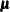, <cov=>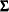)

Multivariate normal distribution with mean vectorand covariance matrixmvnar(<mu=>, sd=, <rho=>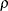)
mvnar(<mu=>, var=, <rho=>)
mvnar(<mu=>, prec=, <rho=>)

Multivariate normal distribution with mean vectorand a covariance matrix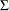. The covariance matrixis a multiple of the scale and a matrix with a first-order autoregressive structure

The following RANDOM statement specifies a scale effect, where s2u can be a constant or a model parameter and index is a data set variable that indicates group membership of the random effect u:

random u ~ normal(0,var=s2u) subject=index;

The following statements specify multidimensional effects, where mu and cov can be either parameters in the model or constant arrays:

array w;
array mu;
array cov[2,2];
random w ~ mvn(mu, cov) subject=index;

You can specify multiple RANDOM statements. Hyperparameters in the prior distribution of a random effect can be other random effects in the model. For example, the following statements are allowed because the random effect g appears in the distribution for the random effect u:

random g ~ normal(0,var=s2g) subject=month;
random u ~ normal(g,var=s2u) subject=day;

These two RANDOM statements specify a nested hierarchical model in which the random-effects g is the hyperparameter of the random-effects u. You can build the hierarchical structure as deep as you want. You can also use multiple RANDOM statements to build non-nested random-effects models, where the effects could enter the model on different levels but not in the same hierarchy of each other.

The number of random-effects parameters in each RANDOM statement is determined by the number of unique values in the SUBJECT= variable, which can be either unsorted numeric or unsorted character literal. Unlike the model parameters that are explicitly declared in the PARMS statement (with therefore a fixed total number), the number of random-effects parameters in a program depends on the values of the SUBJECT= data set variable. That number can change from one BY group to another.

The names of the random-effects parameters are created internally. See the NAMESUFFIX= option for the naming convention of the random-effects parameters. The random-effects parameters are updated conditionally in the simulation. All posterior draws are saved to the OUTPOST= output data set by default, and you can use the MONITOR= option to monitor any of the parameters. For more information about available sampling algorithms, see the ALGORITHM= option. For more information about how to set a random-effects parameter to a constant (also known as corner-point constraint), see the CONSTRAINT option.

You can specify the following options in the RANDOM statement:

ALGORITHM=option
ALG=option

specifies the algorithm to use to sample the posterior distribution. The following options are available:

RWM

uses the random-walk Metropolis algorithm with normal proposal.

SLICE

uses the slice sampling algorithm.

GEO

uses the discrete random-walk Metropolis with symmetric geometric proposal.

When possible, PROC MCMC samples directly from the full conditional distribution. Otherwise, the default sampling algorithm is the RWM.

CONSTRAINT(VALUE=value) = FIRST | LAST | NONE | ’formatted-value
ZERO=FIRST | LAST | NONE | ’formatted-value

sets one of the random-effects parameters to a fixed value. The default is ZERO=NONE, which does not fix any of the parameters to be a constant. This option enables you to eliminate one of the parameters.

For example, this option could be useful if you want to fit a regression model with categorical covariates and, instead of creating a design matrix, you treat the parameters as “random effects” and fit an equivalent random-effects model.

Suppose you have a regression that includes a categorical variable X with J levels. You can construct a full-rank design matrix with J–1 dummy variables (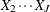with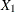being the base group) and fit a regression such as the following: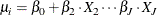The following statements in a PROC MCMC step fit such a hypothetical regression model:

parms beta0 betax2 ... betaxJ;
prior beta: ~ n(0, sd=100);
mu = beta0 + betax2 * x2 + ... betaxJ * xJ;
...

Equivalently, you can also treat this model as a random-effects model such as the following, where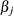are random effects for each category in X: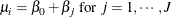However, this random-effects model is over-parameterized. The ZERO= option rids the model with one random-effects parameter of choice and fixes it to be zero. The following example statements fit such a hypothetical random-effects model:

parms beta0;
prior beta0 ~ n(0, sd=100);
random beta ~ n(0, sd=100) subject=x zero=first;
mu = beta0 + beta;
...

The specification ZERO=FIRST sets the first random-effects parameter to 0, implying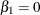. This random-effects parameter corresponds to the first category in the SUBJECT= variable. The category is what the first observation of the SUBJECT= variable takes.

The specification ZERO=LAST sets the last random-effects parameter to be 0, implying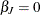. This random-effects parameter corresponds to the last category in the SUBJECT= variable. The category is not necessarily the same category that the last observation of the SUBJECT= variable takes because the SUBJECT= variable does not need to be sorted.

The specification ZERO='formatted-value' sets the random-effects parameter for the category (in the SUBJECT= variable) with a formatted value that matches 'formatted-value' to 0. For example, ZERO='3' sets.

The CONSTRAINT(VALUE=value) option works similarly to the ZERO= option. You can assign an arbitrary value to any one of the random-effects parameter. For example, the specification CONSTRAINT(VALUE=0)=FIRST is equivalent to ZERO=FIRST.

INITIAL=SAS-data-set | constant | numeric-list

specifies the initial values of the random-effects parameters. By default, PROC MCMC uses the same option as specified in the INIT= option to generate initial values for the random-effects parameter: either it uses the mode of the prior density or it randomly draws a sample from that distribution. You can start the Markov chain at different places by providing a SAS-data-set, a constant, or a numeric-list for multivariate random-effects parameters.

If you use a SAS-data-set, the data set must consist of variable names that agree with the random-effects parameters in the model (see the NAMESUFFIX= option for the naming convention of the random-effects parameters). The easiest way to find the names of the internally created parameter names is to run a default analysis with a very small number of simulations and check the variable names in the OUTPOST= data set. You can provide a subset of the initial values in the SAS-data-set and PROC MCMC will use the default mechanism to fill in the rest of the random-effects parameters.

For example, the following statement creates a data set with initial values for the random-effects parameters u_1, u_2, and u_3:

data RandomInit;
input u_1 u_2 u_3;
datalines;
2.3 3 -3
;

The following RANDOM statement takes the values in the RandomInit data set to be the initial values of the corresponding random-effects parameters in the model:

random u ~ normal(0,var=s2u) subject=index init=randominit;

Specifying a constant assigns that constant as the initial value to all random-effects parameters in the statement. For example, the following statement assigns the value 5 to be used as an initial value for all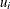in the model:

random u ~ normal(0,var=s2u) subject=index init=5;

If you have multiple effects, you can provide a list of numbers, where the length of the list the same as the dimension of your random-effects array. Each number is then given to all corresponding random-effects parameters in order. For example, the following statement assigns the value 2 to be used as an initial value for all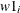and the value 3 to be used for all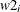in the model:

array w w1 w2;
random w ~ mvn(mu, cov) subject=index init=(2 3);

If you use the GENERAL or DGENERAL functions in the RANDOM statement, you must provide initial values for these parameters.

MONITOR= (symbol-list | number-list | RANDOM(number))

outputs analysis for selected random-effects parameters. You can choose to monitor the random-effects parameters by listing the effect names or effect indices, or you can have them randomly selected by PROC MCMC.

To monitor all random-effects parameters, you specify the effect name in the MONITOR= option:

random u ~ normal(0,var=s2u) subject=index monitor=(u);

You have three options for monitoring a subset of the random-effects parameters. You can provide a list of the parameter names, you can provide a number list of the parameter indices, or you can have PROC MCMC randomly choose a subset of parameters for you.

For example, if you want to monitor analysis for parameters u_1 through u_10, u_23, and u_57, you can provide the names as follows:

random u ~ normal(0,var=s2u) subject=index monitor=(u_1-u_10 u_23 u_57);

The naming convention in the symbol-list must agree with the NAMESUFFIX= option, which controls how the parameter names of the random-effect are created. By default, NAMESUFFIX=SUBJECT, and the symbol-list must use suffixes that correspond to the formatted values in the SUBJECT= data set variable. With the NAMESUFFIX=POSITION option, the symbol-list must use suffixes that agree with the input order of the SUBJECT= variable. If the SUBJECT= variable has a character value, you cannot use the hyphen (-) in the symbol-list to indicate a range of variables.

To monitor the same list of random-effects parameters, you can provide their indices:

random u ~ normal(0,var=s2u) subject=index monitor=(1 to 10 by 1 23 57);

PROC MCMC can also randomly choose a subset of the parameters to monitor:

random u ~ normal(0,var=s2u) subject=index monitor=(random(12));

The sequence of the random indices is controlled by the SEED= option in the PROC MCMC statement.

By default, PROC MCMC does not monitor any random-effects parameters. When you specify this option, it takes the specification of the STATISTICS= and PLOTS= options in the PROC MCMC statement. By default, PROC MCMC outputs all the posterior samples of all random-effects parameters to the OUTPOST= output data set. You can use the NOOUTPOST option to suppress the saving of the random-effects parameters.

NAMESUFFIX=option

specifies how the names of the random-effects parameters are internally created from the SUBJECT= variable that is specified in the RANDOM statement. PROC MCMC creates the names by concatenating the random-effect symbol with an underscore and a series of numbers or characters. The following options control the type of methods that are used in such contruction:

SUBJECT

constructs the parameter names by appending the formatted values of the SUBJECT= variable in the input data setfnREnames.

POSITION

constructs the parameter names by appending the numbers 1, 2, 3, and so on, where the number indicates the order in which the SUBJECT= variable appears in the data set.

For example, suppose you have an input data set with four observations and the SUBJECT= variable zipcode has four values (with three of them unique): 27513, 01440, 27513, and 15217. The following SAS statement creates three random-effects parameters named u_27513, u_01440, and u_15217:

random u ~ normal(0,var=10) subject=zipcode namesuffix=subject;

On the other hand, using NAMESUFFIX=POSITION creates three parameters named as u_1, u_2, and u_3:

random u ~ normal(0,var=10) subject=zipcode namesuffix=position;

By default, NAMESUFFIX=SUBJECT.

NOOUTPOST

suppresses the output of the posterior samples of random-effects parameters to the OUTPOST= data set. In models with a large number of random-effects parameters (for example, tens of thousands), PROC MCMC can run faster if it does not save the posterior samples of the random-effects parameters.

When you specify both the NOOUTPOST option and the MONITOR= option, PROC MCMC outputs the list of variables that are monitored.

The maximum number of variables that can be saved to an OUTPOST= data set is 32,767. If you run a large-scale random-effects model with the number of parameters exceeding the limit, the NOOUTPOST option is evoked automatically and PROC MCMC does not save the random-effects parameter draws to the posterior output data set. You can use the MONITOR= option to select a subset of the parameters to store in the OUTPOST= data set.

 In SAS/STAT 9.3, the random-effects parameters were created using the unformatted values of the SUBJECT= variable.